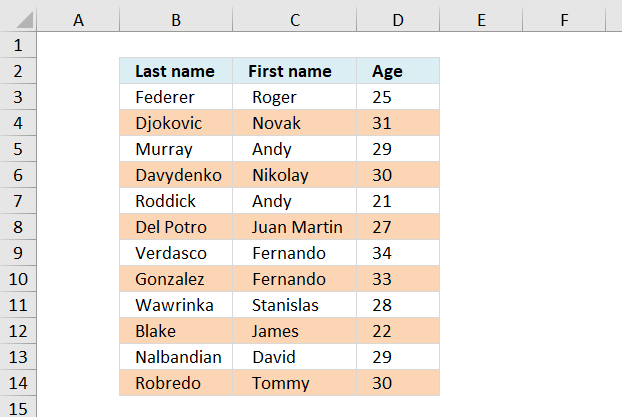Author: Oscar Cronquist Article last updated on October 13, 2018Here is how to highlight every other row using conditional formatting.

Conditional formatting formula:

=ISEVEN(ROW())*OR(\$B3:\$D3<>"")

Alternative CF formula:

=EVEN(ROW())=ROW()

This formula colors every second row if any cell in the row is populated. If you know you will add more records later to the list, select and conditional format a range larger than the list. Empty rows won´t be formatted.

How to apply the conditional formatting formula in excel 2007:

1. Select the range, example cell range A:C
2. Click "Home" tab on the ribbon
3. Click "Conditional formatting"
4. Click "New rule..."
5. Click "Use a formula to determine which cells to format"
6. Click "Format values where this formula is true" window.
7. Type =ISEVEN(ROW())*OR(\$B3:\$D3<>"")
8. Click Format button
9. Click "Fill" tab
10. Select a color
11. Click OK!
12. Click OK!

### Explaining the contional formatting formula in cell A2

=ISEVEN(ROW())*OR(\$A2:\$C2<>"")

Step 1 - Check if row number is even

=ISEVEN(ROW())*OR(\$A2:\$C2<>"")

ISEVEN(ROW())

becomes

ISEVEN(2)

returns TRUE in cell A2.

Step 2 - Check if  any of the cells in row are not empty

=ISEVEN(ROW())*OR(\$A2:\$C2<>"")

OR(\$A2:\$C2<>"")

becomes

OR({"Federer", " Roger ", 25}<>"")

becomes

OR({TRUE, TRUE, TRUE})

and returns TRUE

Step 3 - Multiply functions (AND logic)

Both functions need to return TRUE in order to return TRUE

=ISEVEN(ROW())*OR(\$A2:\$C2<>"")

becomes

TRUE*TRUE

and returns TRUE. Row 2 is conditional formatted.

### Download excel sample file for this tutorial

color-every-second-row-using-dynamic-conditional-formatting.xls
(Excel 97-2003 Workbook *.xls)
Functions used in this article

OR(logical1, logical2, …)
Checks whether any of the arguments are TRUE and returns TRUE or FALSE. Returns FALSE only if all arguments are FALSE

ROW(reference)
Returns the row number of a reference

ISEVEN(number)
Returns TRUE if the number is even